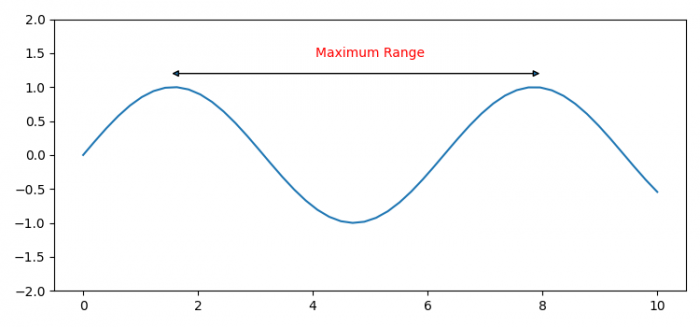# How to annotate a range of the X-axis in Matplotlib?

To annotate a range of the X-axis in matplotlib, we can take the following steps −

• Set the figure size and adjust the padding between and around the subplots.
• Create xx and yy data points using numpy.
• Create a figure and a set of subplots.
• Plot xx and yy data points using plot() method.
• Set ylim of the axis.
• Use annotate method to place arrow heads and range tag name.
• To display the figure, use show() method.

## Example

import matplotlib.pyplot as plt
import numpy as np

plt.rcParams["figure.figsize"] = [7.50, 3.50]
plt.rcParams["figure.autolayout"] = True

xx = np.linspace(0, 10)
yy = np.sin(xx)

fig, ax = plt.subplots(1, 1)

ax.plot(xx, yy)
ax.set_ylim([-2, 2])

ax.annotate('', xy=(5,2), xytext=(8,2),
xycoords='data', textcoords='data',
arrowprops={'arrowstyle': '<|-|>'}, color='yellow')

ax.annotate('Maximum Range', xy=(5,5), ha='center', va='center', color='red')

plt.show()

## Output